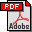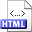The region approach for computing relative neighbourhood graphs in the Lp metric
Author:Jyrki Katajainen
Published in:Computing 40,2 (1988), 147-161
Full text:PDF (793 kB)
DOI:10.1007/BF02247943
Abstract:The following geometrical proximity concepts are discussed: relative closeness and geographic closeness. Consider a set V = {v1,v2,...,vn} of distinct points in a two-dimensional space. The point vj is said to be a relative neighbour of vi if
 dp(vi,vj) ≤ max{dp(vj,vk), dp(vj,vk)} for all vk ∈ V,
where dp denotes the distance in the Lp metric, 1 ≤ p ≤ ∞. After dividing the space around the point vi into eight sectors (regions) of equal size, a closest point to vi in some region is called an octant (region, or geographic) neighbour of vi. For any Lp metric, a relative neighbour of vi is always an octant neighbour in some region at vi. This gives a direct method for computing all relative neighbours, i.e. for establishing the relative neighbourhood graph of V. For every point vi of V, first search for the octant neighbours of vi in each region, and then for each octant neighbour vj found check whether the point vj is also a relative neighbour of vi. In the Lp metric, 1 < p < ∞, the total number of octant neighbours is shown to be Θ(n) for any set of n points; hence, even a straightforward implementation of the above method runs in Θ(n2) time. In the L1 and L metrics the method can be refined to a Θ(n log n + m) algorithm, where m is the number of relative neighbours in the output, n − 1 ≤ mn (n − 1). The L1 (L) algorithm is optimal within a constant factor.
Related:HTML (Thesis)
BibLATEX:
```@article{Kat1988J,
author = {Jyrki Katajainen},
title = {The region approach for computing relative neighbourhood graphs in
the \$L_p\$ metric},
journaltitle = {Computing},
volume = {40},
number = {2},
year = {1988},
pages = {147--161},
}
```